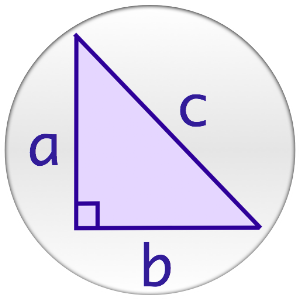## Pythagorean Theorem Calculator helps you practice Grade 8 Math - Lumos Educational App StorePrice -

#### DESCRIPTION:

The Pythagorean Theorem can be difficult to solve by hand. This app was created to find the missing side of a right triangle using the Pythagorean Theorem! You simply need to enter the two...

#### OVERVIEW:

Pythagorean Theorem Calculator is a free educational mobile app By .It helps students in grades 8 practice the following standards 8.G.B.6,8.G.B.7,8.G.B.8.

This page not only allows students and teachers download Pythagorean Theorem Calculator but also find engaging Sample Questions, Videos, Pins, Worksheets, Books related to the following topics.

1. 8.G.B.6 : Explain a proof of the Pythagorean Theorem and its converse. .

2. 8.G.B.7 : Apply the Pythagorean Theorem to determine unknown side lengths in right triangles in real-world and mathematical problems in two and three dimensions..

3. 8.G.B.8 : Apply the Pythagorean Theorem to find the distance between two points in a coordinate system..

8

#### STANDARDS:

8.G.B.6
8.G.B.7
8.G.B.8

Software Version:

Category:

### RELATED APPS

Rate this App?
0

0 Ratings & 0 Reviews

5
0
0
4
0
0
3
0
0
2
0
0
1
0
0EdSearch WebSearch